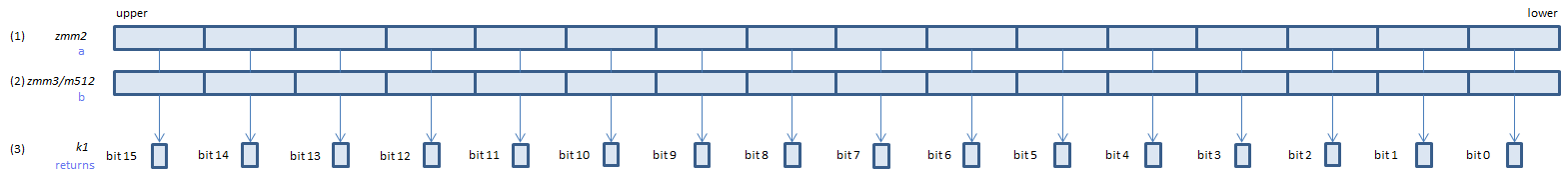﻿ vcmptrue_usps

## VCMPTRUE_USPS - CoMPare TRUE Unordered Signal Packed Single

VCMPTRUE_USPS xmm1, xmm2, xmm3/m128    (V1
__m128 _mm_cmp_ps(__m128 a, __m128 b, _CMP_TRUE_US)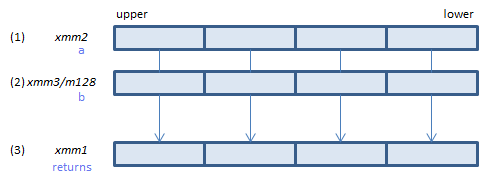For each float, always set 1 to all bits of the corresponding float of (3).
VCMPTRUE_USPS ymm1, ymm2, ymm3/m256    (V1
__m256 _mm256_cmp_ps(__m256 a, __m256 b, _CMP_TRUE_US)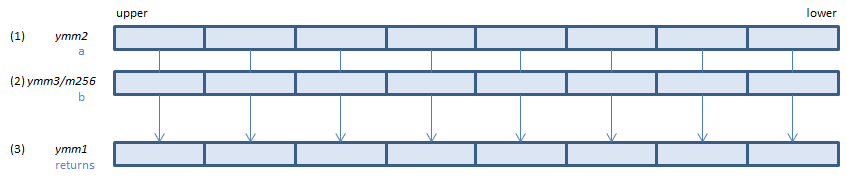For each float, always set 1 to all bits of the corresponding float of (3).
VCMPTRUE_USPS k1{k2}, xmm2, xmm3/m128/m32bcst    (V5+VL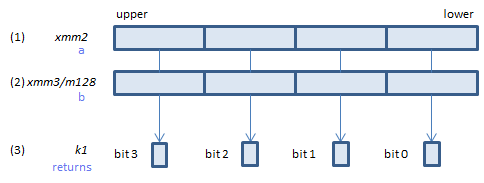For each float, always set 1 to the corresponding bit of (3).
If k2 bit is 0, the comparison is not done and the corresponding bit of (3) is set to zero. Upper bits of (3) are zero cleared.
VCMPTRUE_USPS k1{k2}, ymm2, ymm3/m256/m32bcst    (V5+VL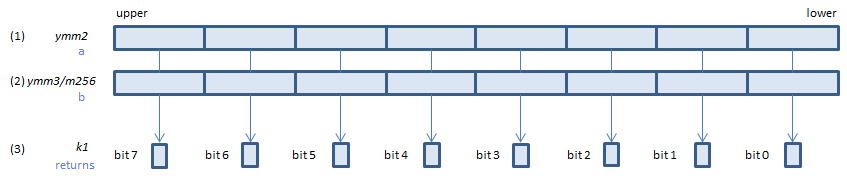For each float, always set 1 to the corresponding bit of (3).
If k2 bit is 0, the comparison is not done and the corresponding bit of (3) is set to zero. Upper bits of (3) are zero cleared.
VCMPTRUE_USPS k1{k2}, zmm2, zmm3/m512/m32bcst{sae}    (V5